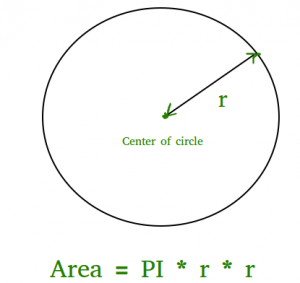GeeksforGeeks App
Open AppBrowser
Continue

# Program to find area of a circle

Given the radius of a circle, find the area of that circle.

The area of a circle can simply be evaluated using the following formula.where r is radius of circle and it maybe in float because value of pie is 3.14

Approach: Using the given radius, find the area using the above formula: (pi * r * r) and print the result in float.

## C++

 // C++ program to find area// of circle#include const double pi = 3.14159265358979323846;using namespace std;  // function to calculate the area of circlefloat findArea(float r){    return (pi * r * r);}// driver codeint main(){    float r, Area;    r = 5;      // function calling    Area = findArea(r);    // displaying the area    cout << "Area of Circle is :" << Area;    return 0;}

## C

 // C program to find area// of circle#include #include #define PI 3.142  double findArea(int r){   return PI * pow(r, 2);}  int main(){    printf("Area is %f", findArea(5));    return 0;}

## Java

 // Java program to find area// of circle      class Test{    static final double PI = Math.PI;               static double findArea(int r)    {       return PI * Math.pow(r, 2);    }               // Driver method    public static void main(String[] args)     {        System.out.println("Area is " +  findArea(5));    }}

## Python3

 # Python3 program to find Area of a circle  def findArea(r):    PI = 3.142    return PI * (r*r);  # Driver methodprint("Area is %.6f" % findArea(5));  # This code is contributed by Chinmoy Lenka

## C#

 // C# program to find area of circleusing System;  class GFG{    static double PI = Math.PI;              static double findArea(int r)    {    return PI * Math.Pow(r, 2);    }              // Driver method    static void Main()     {        Console.Write("Area is " + findArea(5));    }}  // This code is contributed by Sam007.

## PHP

 

## Javascript

 

Output

Area of Circle is :78.5398

Time Complexity: O(1)
Auxiliary Space: O(1), since no extra space has been taken.

My Personal Notes arrow_drop_up